# Common Core: High School - Geometry : Prove Triangle Theorems: CCSS.Math.Content.HSG-CO.C.10

## Example Questions

← Previous 1 3

### Example Question #1 : Prove Triangle Theorems: Ccss.Math.Content.Hsg Co.C.10

Are the two triangles shown congruent?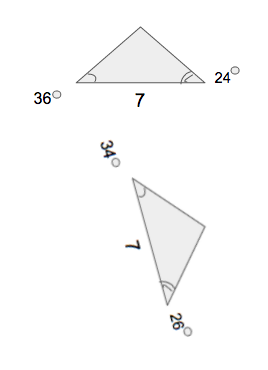No

Yes

Yes

Explanation:

For two triangles to be congruent they must have equal corresponding angles and sides. There are five geometric theorems that can be used to prove whether triangles are congruent or not. Since, these two triangles have two defined angles and the side between the angles are defined as well, the Angle, Side, Angle geometric theorem can be used.Looking at the triangles the corresponding angles are equal and the correspond side is also equal therefore, the triangles are congruent.

### Example Question #1 : Prove Triangle Theorems: Ccss.Math.Content.Hsg Co.C.10

Are the two triangles congruent?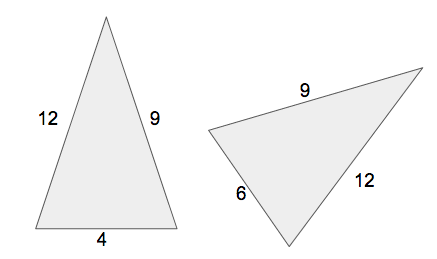Yes

No

Yes

Explanation:

For two triangles to be congruent they must have equal corresponding angles and sides. There are five geometric theorems that can be used to prove whether triangles are congruent or not. Since, these two triangles have all three sides defined, the Side, Side, Side geometric theorem can be used.Looking at the triangles the corresponding sides are equal therefore, the triangles are congruent.

### Example Question #1 : Prove Triangle Theorems: Ccss.Math.Content.Hsg Co.C.10

Are the two triangles congruent?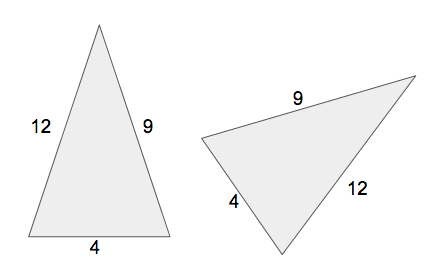No

Yes

Yes

Explanation:

For two triangles to be congruent they must have equal corresponding angles and sides. There are five geometric theorems that can be used to prove whether triangles are congruent or not. Since, these two triangles have all three sides defined, the Side, Side, Side geometric theorem can be used.Looking at the triangles the corresponding sides are equal therefore, the triangles are congruent.

### Example Question #2 : Prove Triangle Theorems: Ccss.Math.Content.Hsg Co.C.10

Are the two triangles congruent?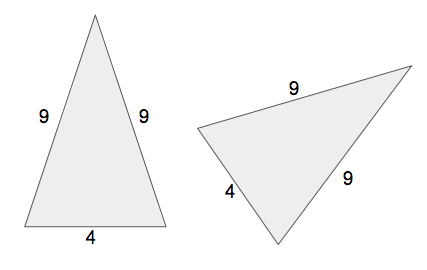No

Yes

Yes

Explanation:

For two triangles to be congruent they must have equal corresponding angles and sides. There are five geometric theorems that can be used to prove whether triangles are congruent or not. Since, these two triangles have all three sides defined, the Side, Side, Side geometric theorem can be used.Looking at the triangles the corresponding sides are equal therefore, the triangles are congruent.

### Example Question #2 : Prove Triangle Theorems: Ccss.Math.Content.Hsg Co.C.10

Are the two triangles shown congruent?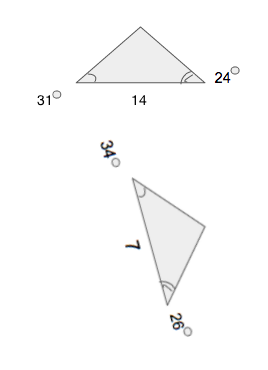No

Yes

No

Explanation:

For two triangles to be congruent they must have equal corresponding angles and sides. There are five geometric theorems that can be used to prove whether triangles are congruent or not. Since, these two triangles have two defined angles and the side between the angles are defined as well, the Angle, Side, Angle geometric theorem can be used.Looking at the triangles the corresponding angles are not equal and the correspond side is also not equal therefore, the triangles are not congruent.

### Example Question #2 : Prove Triangle Theorems: Ccss.Math.Content.Hsg Co.C.10

Are the two triangles congruent?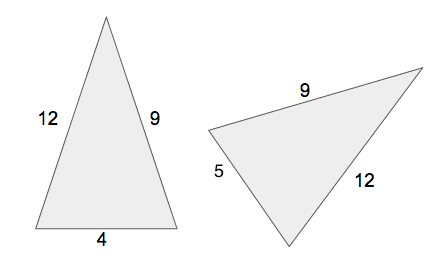Yes

No

No

Explanation:

For two triangles to be congruent they must have equal corresponding angles and sides. There are five geometric theorems that can be used to prove whether triangles are congruent or not. Since, these two triangles have all three sides defined, the Side, Side, Side geometric theorem can be used.Looking at the triangles the corresponding sides are not equal therefore, the triangles are not congruent.

### Example Question #91 : High School: Geometry

Are the two triangles shown congruent?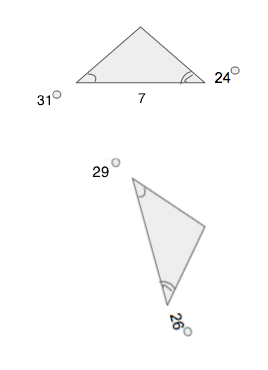No

Yes

No

Explanation:

For two triangles to be congruent they must have equal corresponding angles and sides. There are five geometric theorems that can be used to prove whether triangles are congruent or not. Since, these two triangles have two defined angles and the side between the angles are defined as well, the Angle, Side, Angle geometric theorem can be used.Looking at the triangles the corresponding angles are not equal therefore, the triangles are not congruent.

### Example Question #92 : High School: Geometry

Are the two triangles shown congruent?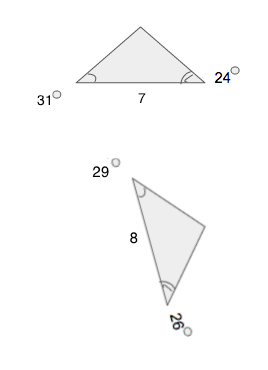Yes

No

No

Explanation:

For two triangles to be congruent they must have equal corresponding angles and sides. There are five geometric theorems that can be used to prove whether triangles are congruent or not. Since, these two triangles have two defined angles and the side between the angles are defined as well, the Angle, Side, Angle geometric theorem can be used.Looking at the triangles the corresponding angles are not equal and the correspond side is also not equal therefore, the triangles are not congruent.

### Example Question #93 : High School: Geometry

Are the two triangles congruent?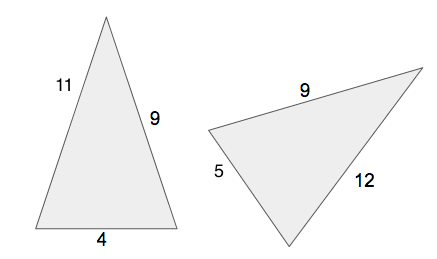No

Yes

No

Explanation:

For two triangles to be congruent they must have equal corresponding angles and sides. There are five geometric theorems that can be used to prove whether triangles are congruent or not. Since, these two triangles have all three sides defined, the Side, Side, Side geometric theorem can be used.Looking at the triangles the corresponding sides are not equal therefore, the triangles are not congruent.

### Example Question #11 : Prove Triangle Theorems: Ccss.Math.Content.Hsg Co.C.10

Are the two triangles congruent?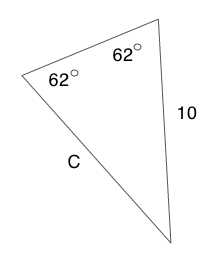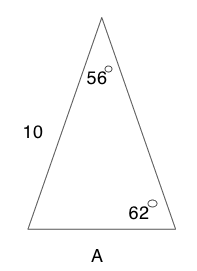Yes

No

Yes

Explanation:

For two triangles to be congruent they must have equal corresponding angles and sides. There are five geometric theorems that can be used to prove whether triangles are congruent or not. Since, these two triangles have two defined angles and the side defined as well, the Angle, Angle, Side geometric theorem can be used.Looking at the triangles the corresponding angles are equal and the correspond side is also equal therefore, the triangles are congruent.

← Previous 1 3

### All Common Core: High School - Geometry Resources